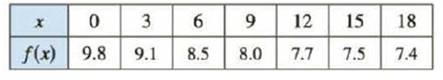Chapter 7.7, Problem 40E

Chapter
Section
Textbook Problem

The table shows values of a force function f(x), where x is measured in meters and f(x) in newtons. Use Simpson’s Rule to estimate the work done by the force in moving an object a distance of 18 m.To determine

To Estimate: The work done by the force in moving an object a distance of 18 m by using Simpson’s rule.

Explanation

Given information:

The force function f(x) with respect to distance x is shown in table.

The function f(x) measured in Newton’s and x is measured in meters.

The upper limit is 18 and lower limit is 0.

The number of sub intervals as 6 (n=6).

Calculation:

Express the work done by the force in moving an object a distance of 18 m is as follows:

018f(x)dx (1)

Show the Simpson’s rule as follows:

abf(x)dxSn=Δx3[f(x0)+4f(x1)+2f(x2)+4f(x3)...+2f(xn2)+4f(xn1)+f(xn)]

Consider the condition for Simpson rule as follows:

• Δx=ban
•  Number of subinterval n is even.

Here, Sn is Simpson’s approximation, x1,x2,...xn are subintervals, b is upper limit, a is lower limit, and n is number of subintervals.

Calculate the length of the subinterval (Δx) using the formula:

Δx=ban

Here, b is upper limit, a is lower limit, and n is number of subintervals.

Substitute 18 for b, 0 for a, and 6 for n.

Δx=1806=3

Hence, the subinterval length is 3 for the limits [0,18].

The force function has each 3 m intervals. According to table values, the subinterval values are 0, 3, 6, 9, 12, 15, and 18 for x0, x1, x2, x3, x4, x5, and x6.

Calculate the work done by the force using Simpson’s rule.

Apply Simpson’s rule in Equation (1).

Substitute 3 for Δx and 6 for n.

018f(x)dx=S6=33[f(x0)+4f(x1)+2f(x2)+4f(x3)+2f(x4)+4f(x5)+f(x6)] (2)

Apply the subinterval values in Equation (2)

Still sussing out bartleby?

Check out a sample textbook solution.

See a sample solution

The Solution to Your Study Problems

Bartleby provides explanations to thousands of textbook problems written by our experts, many with advanced degrees!

Get Started

In Exercises 1520, simplify the expression. 16. a6b5(a3b2)3

Applied Calculus for the Managerial, Life, and Social Sciences: A Brief Approach

Solve the equations in Exercises 112 for x (mentally, if possible). x+5=0

Finite Mathematics and Applied Calculus (MindTap Course List)

Polynomials Find the sum, difference, or product. 15. (12x 7) (5x 12)

Precalculus: Mathematics for Calculus (Standalone Book)